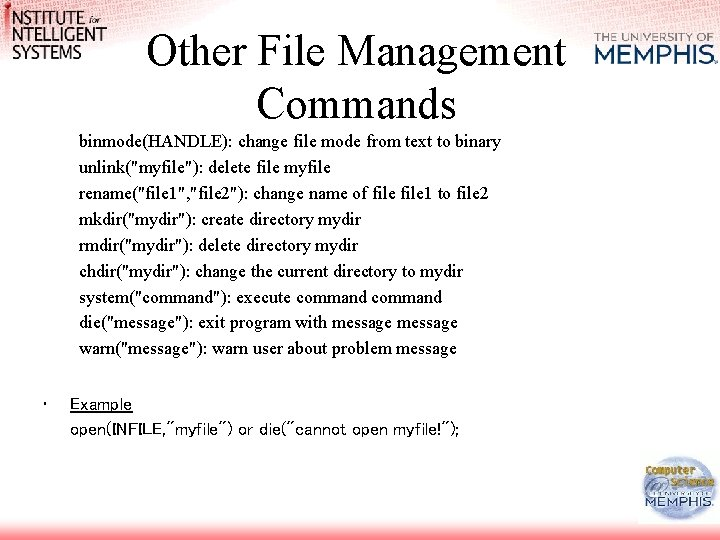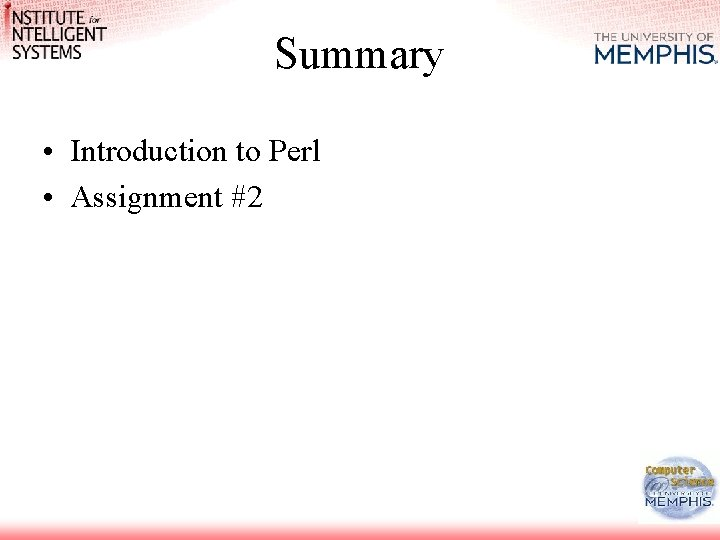# Information Retrieval and Web Search Vasile Rus Ph

• Slides: 48Information Retrieval and Web Search Vasile Rus, Ph. D [email protected] edu www. cs. memphis. edu/~vrus/teaching/irwebsearch/Outline • Announcements • Introduction to Perl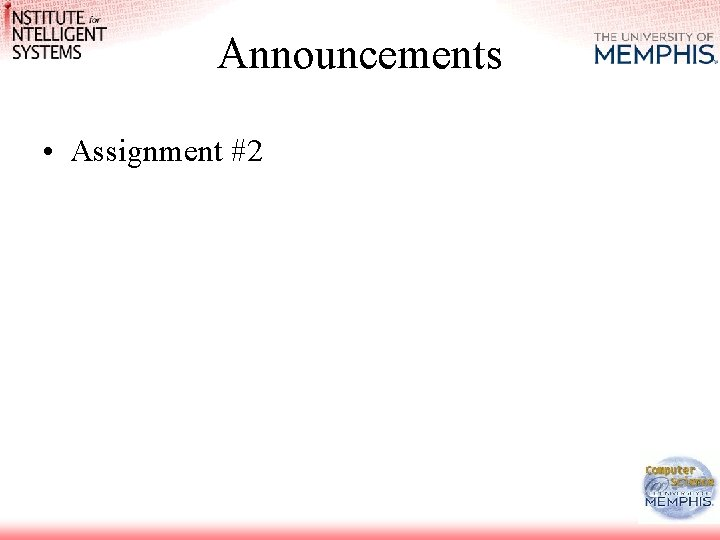Announcements • Assignment #2About Perl • 1987 – Larry Wall Develops PERL • 1989 – October 18 Perl 3. 0 is released under the GNU Protection License • 1991 – March 21 Perl 4. 0 is released under the GPL and the new Perl Artistic License • Now • Perl 6 PERL is not officially a Programming Language per se. Wall’s original intent was to develop a scripting language more powerful than Unix Shell Scripting, but not as tedious as C. PERL is an interpreted language. That means that there is no explicitly separate compilation step. Rather, the processor reads the whole file, converts it to an internal form and executes it immediately. P. E. R. L. = Practical Extraction and Report Language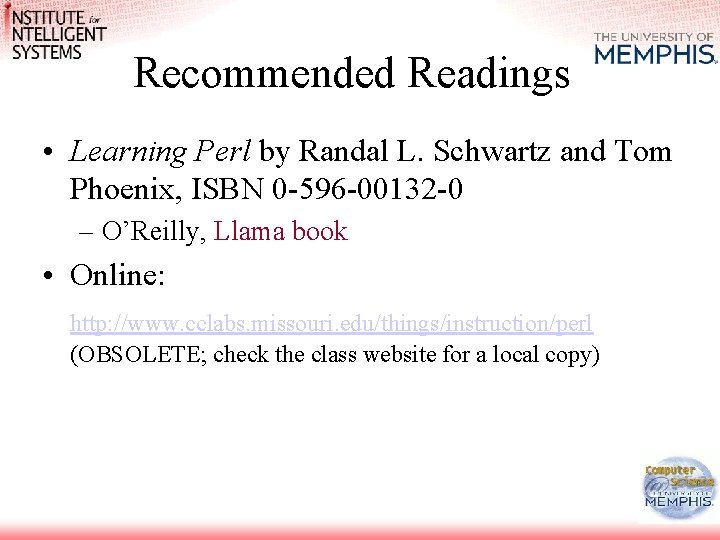Recommended Readings • Learning Perl by Randal L. Schwartz and Tom Phoenix, ISBN 0 -596 -00132 -0 – O’Reilly, Llama book • Online: http: //www. cclabs. missouri. edu/things/instruction/perl (OBSOLETE; check the class website for a local copy)Taste of Perl • Problem: Substitute Text In Multiple Files perl -e 's/andrew/andy/gi' -p -i. bak *. txt – g: globally – i: ignoring cases • next slide presents a procedural equivalent to the same problem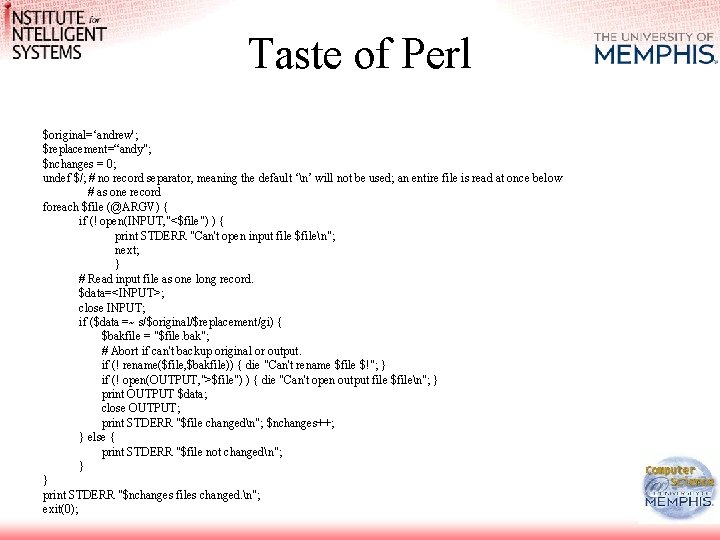Taste of Perl \$original=‘andrew'; \$replacement=“andy"; \$nchanges = 0; undef \$/; # no record separator, meaning the default ‘n’ will not be used; an entire file is read at once below # as one record foreach \$file (@ARGV) { if (! open(INPUT, "<\$file") ) { print STDERR "Can't open input file \$filen"; next; } # Read input file as one long record. \$data=<INPUT>; close INPUT; if (\$data =~ s/\$original/\$replacement/gi) { \$bakfile = "\$file. bak"; # Abort if can't backup original or output. if (! rename(\$file, \$bakfile)) { die "Can't rename \$file \$!"; } if (! open(OUTPUT, ">\$file") ) { die "Can't open output file \$filen"; } print OUTPUT \$data; close OUTPUT; print STDERR "\$file changedn"; \$nchanges++; } else { print STDERR "\$file not changedn"; } } print STDERR "\$nchanges files changed. n"; exit(0);Variables • A variable is a name of a place where some information is stored. For example: \$year. Of. Birth = 1976; \$current. Year = 2000; \$age = \$current. Year-\$year. Of. Birth; print \$age; • The variables in the example program can be identified as such because their names start with a dollar (\$). Perl uses different prefix characters for structure names in programs. Here is an overview: \$: variable containing scalar values such as a number or a string @: variable containing a list with numeric keys %: variable containing a list with strings as keys &: subroutine *: matches all structures with the associated name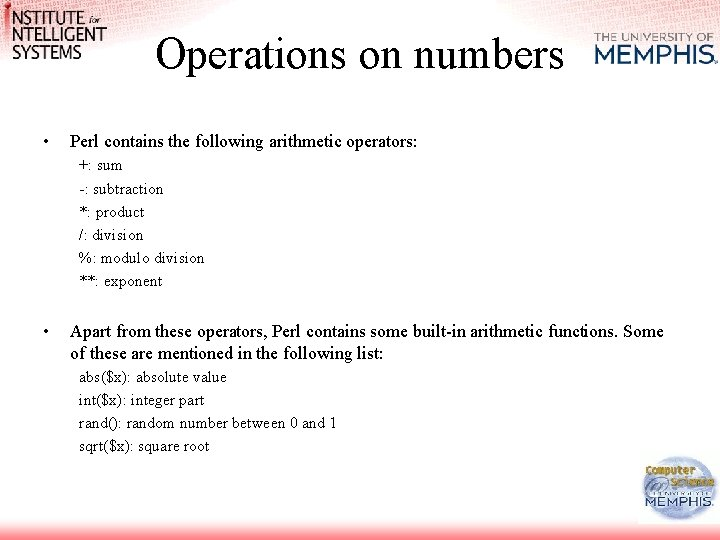Operations on numbers • Perl contains the following arithmetic operators: +: sum -: subtraction *: product /: division %: modulo division **: exponent • Apart from these operators, Perl contains some built-in arithmetic functions. Some of these are mentioned in the following list: abs(\$x): absolute value int(\$x): integer part rand(): random number between 0 and 1 sqrt(\$x): square root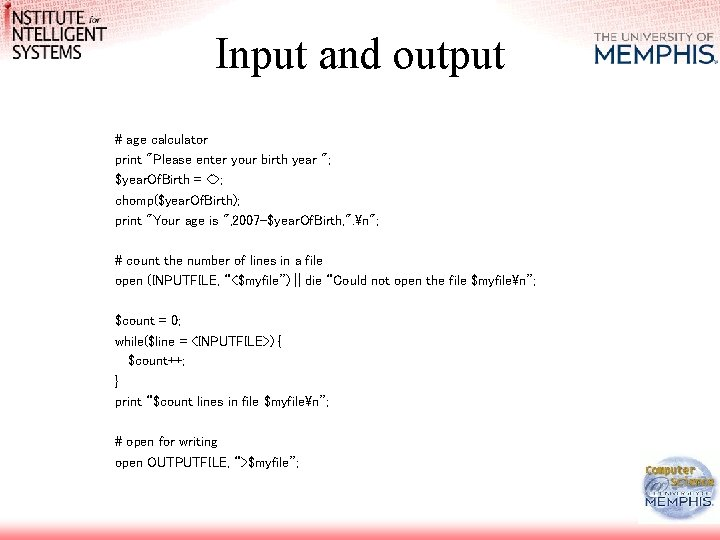Input and output # age calculator print "Please enter your birth year "; \$year. Of. Birth = <>; chomp(\$year. Of. Birth); print "Your age is ", 2007 -\$year. Of. Birth, ". n"; # count the number of lines in a file open (INPUTFILE, “<\$myfile”) || die “Could not open the file \$myfilen”; \$count = 0; while(\$line = <INPUTFILE>) { \$count++; } print “\$count lines in file \$myfilen”; # open for writing open OUTPUTFILE, “>\$myfile”;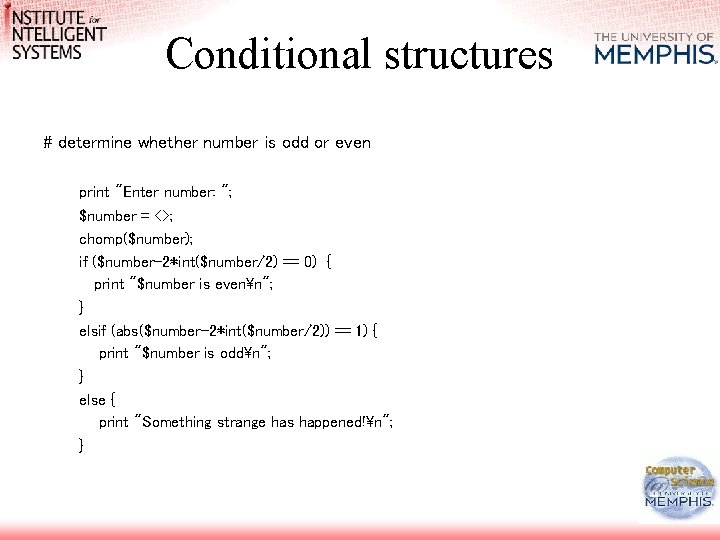Conditional structures # determine whether number is odd or even print "Enter number: "; \$number = <>; chomp(\$number); if (\$number-2*int(\$number/2) == 0) { print "\$number is evenn"; } elsif (abs(\$number-2*int(\$number/2)) == 1) { print "\$number is oddn"; } else { print "Something strange has happened!n"; }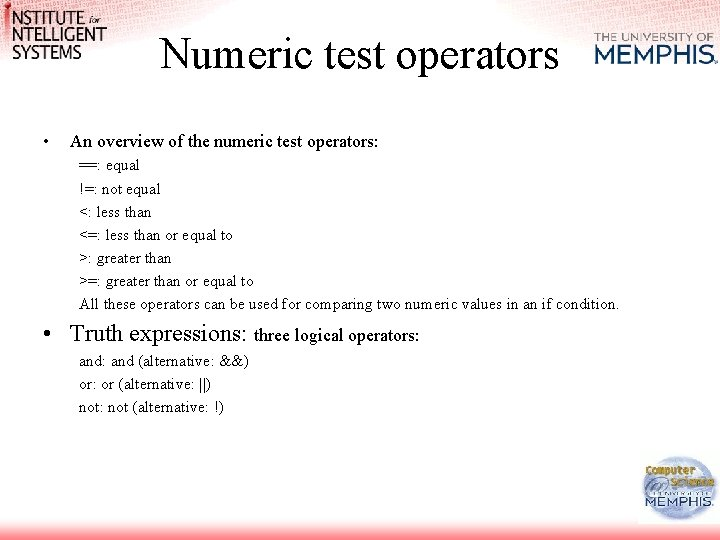Numeric test operators • An overview of the numeric test operators: ==: equal !=: not equal <: less than <=: less than or equal to >: greater than >=: greater than or equal to All these operators can be used for comparing two numeric values in an if condition. • Truth expressions: three logical operators: and (alternative: &&) or: or (alternative: ||) not: not (alternative: !)Iterative structures • #print numbers 1 -10 in three different ways \$i = 1; while (\$i<=10) { print "\$in"; \$i++; } for (\$i=1; \$i<=10; \$i++) { print "\$in"; } foreach \$i (1, 2, 3, 4, 5, 6, 7, 8, 9, 10) { print "\$in"; } • Stop a loop, or force continuation: last; next; • # C break # C continue; Exercise: Read ten numbers and print the largest, the smallest and a count representing how many of them are divisible by three. if (not(defined(\$largest)) or \$number > \$largest) { \$largest = \$number; } if (not(defined(\$smallest)) or \$number < \$smallest) { \$smallest = \$number; } if (\$number-3*int(\$number/3) == 0) { \$count 3++; }A parenthesis: PERL philosophy(ies) • There is more than one way to do it • If you want to shoot yourself in the foot, who am I to stop you? • And a comment: DO write comments in your Perl programs!Basic string operations • • strings are stored in the same type of variables we use for storing numbers string values can be specified between double and single quotes !!! in the former specification variables will be evaluated, in the latter they will not. Comparison operators for strings eq: equal ne: not equal lt: less than le: less than or equal to gt: greater than ge: greater than or equal to - Examples: if (\$a eq \$b) { …. }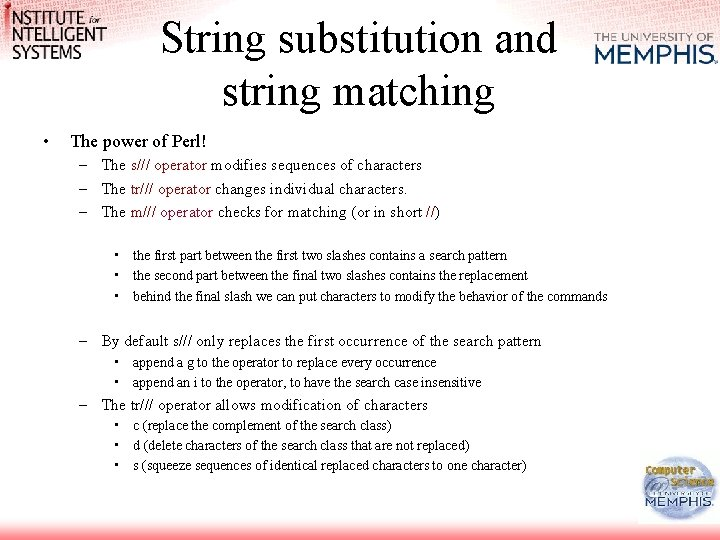String substitution and string matching • The power of Perl! – The s/// operator modifies sequences of characters – The tr/// operator changes individual characters. – The m/// operator checks for matching (or in short //) • the first part between the first two slashes contains a search pattern • the second part between the final two slashes contains the replacement • behind the final slash we can put characters to modify the behavior of the commands – By default s/// only replaces the first occurrence of the search pattern • append a g to the operator to replace every occurrence • append an i to the operator, to have the search case insensitive – The tr/// operator allows modification of characters • c (replace the complement of the search class) • d (delete characters of the search class that are not replaced) • s (squeeze sequences of identical replaced characters to one character)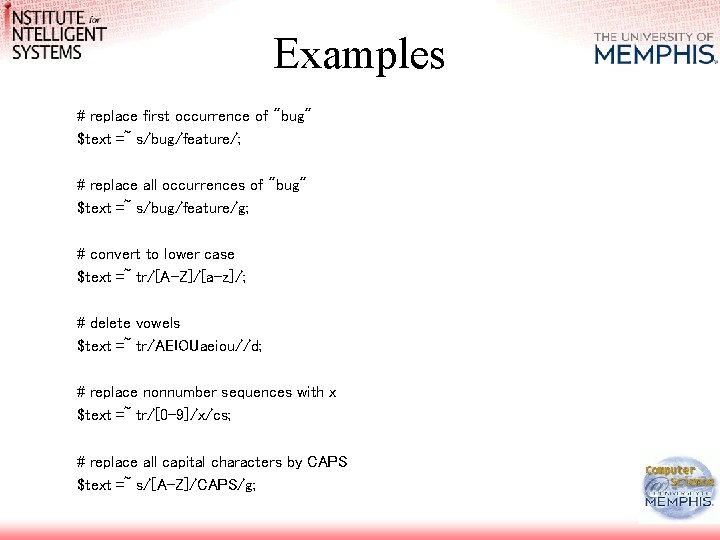Examples # replace first occurrence of "bug" \$text =~ s/bug/feature/; # replace all occurrences of "bug" \$text =~ s/bug/feature/g; # convert to lower case \$text =~ tr/[A-Z]/[a-z]/; # delete vowels \$text =~ tr/AEIOUaeiou//d; # replace nonnumber sequences with x \$text =~ tr/[0 -9]/x/cs; # replace all capital characters by CAPS \$text =~ s/[A-Z]/CAPS/g;Grep with Perl • Simple example: Print all lines from a file that include a given sequence of characters [emulate grep behavior] • Regular Expression: is a template for strings – In Perl a regular expression is like a predicate: • • Returns true if a string matches the regular expression False, otherwise \$_ = “pattern matching”; if ( /pattern/ ){ # equivalent to (\$_ =~ /pattern/) print “matching”; } else { print “not matching”; }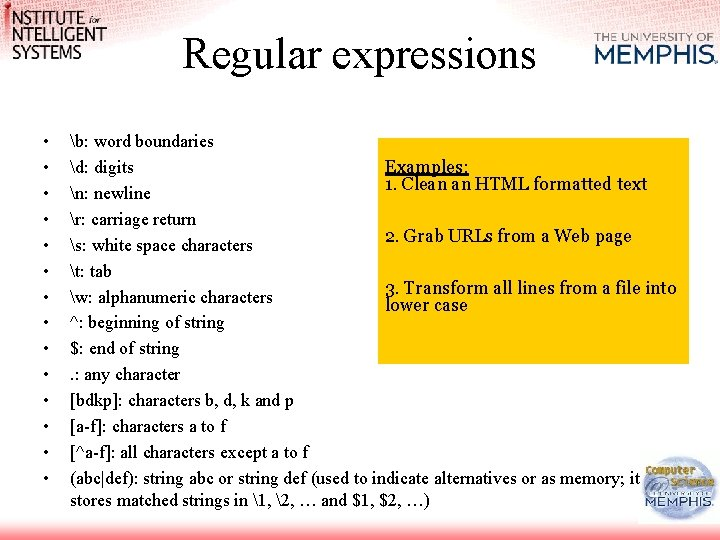Regular expressions • • • • b: word boundaries Examples: d: digits 1. Clean an HTML formatted text n: newline r: carriage return 2. Grab URLs from a Web page s: white space characters t: tab 3. Transform all lines from a file into w: alphanumeric characters lower case ^: beginning of string \$: end of string. : any character [bdkp]: characters b, d, k and p [a-f]: characters a to f [^a-f]: all characters except a to f (abc|def): string abc or string def (used to indicate alternatives or as memory; it stores matched strings in 1, 2, … and \$1, \$2, …)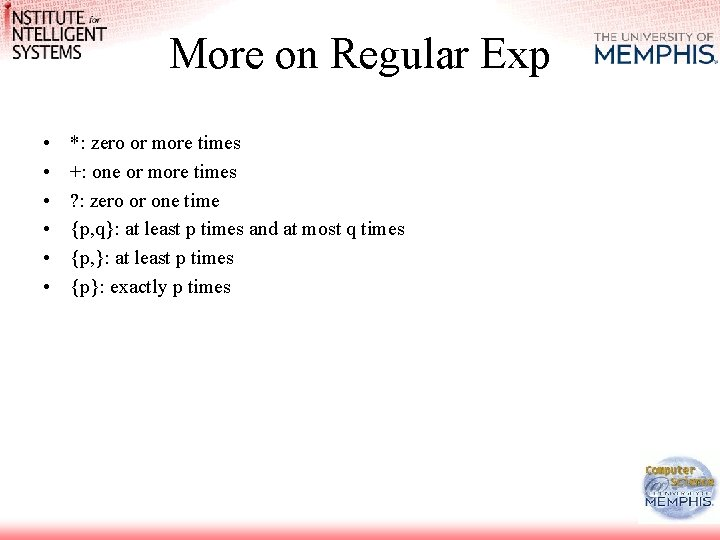More on Regular Exp • • • *: zero or more times +: one or more times ? : zero or one time {p, q}: at least p times and at most q times {p, }: at least p times {p}: exactly p timesLists and Arrays • Scalar: elementary data – numbers or strings (in Perl) • List = ordered collection of scalars • Array = variable that contains a list • Element of a list or array is an independent scalarLists and Arrays @a = (); # empty list @b = (1, 2, 3); # three numbers @c = ("Jan", "Piet", "Marie"); # three strings @d = ("Dirk", 1. 92, 46, "20 -03 -1977"); # a mixed list Variables and sublists are interpolated in a list @b = (\$a, \$a+1, \$a+2); # variable interpolation, assume \$a = 1 @c = ("Jan", ("Piet", "Marie")); # list interpolation @d = ("Dirk", 1. 92, 46, (), "20 -03 -1977"); # empty list interpolation @e = ( @b, @c ); # same as (1, 2, 3, "Jan", "Piet", "Marie") Practical construction operators (\$x. . \$y) @x = (1. . 6) # same as (1, 2, 3, 4, 5, 6) @y = (1. 2. . 5. 2) # same as (1. 2, 2. 2, 3. 2, 4. 2, 5. 2) @z = (2. . 5, 8, 11. . 13) # same as (2, 3, 4, 5, 8, 11, 12, 13) qw() ("quote word") function qw(Jan Piet Marie) is a shorter notation for ("Jan", "Piet", "Marie").Split function \$string = "Jan Pietn. Marie t. Dirk"; @list = split /s+/, \$string; # yields ( "Jan", "Piet", "Marie", "Dirk" ) \$string = " Jan Pietn. Marie t. Dirkn"; # watch out, empty string at the begin and end!!! @list = split /s+/, \$string; # yields ( "", "Jan", "Piet", "Marie", "Dirk", "" ) \$string = "Jan: Piet; Marie---Dirk"; # use any regular expression. . . @list = split /[: ; ]|---/, \$string; # yields ( "Jan", "Piet", "Marie", "Dirk" ) \$string = "Jan Piet"; # use an empty regular expression to split on letters @letters= split //, \$string; # yields ( "J", "a", "n", "P", "i", "e", "t")Split Function - Example: 1. Separate simple punctuation from words in a text (, . ; ! ? ( ) ) 2. Add all the digits in a numberMore about Arrays @array = ("an", "bert", "cindy", "dirk"); \$length = @array; # \$length now has the value 4 @array = ("an", "bert", "cindy", "dirk"); \$length = @array; print \$length; # prints 4 print \$#array; # prints 3 print \$array[\$#array] # prints "dirk" print scalar(@array) # prints 4More about Arrays (\$a, \$b) = ("one", "two"); (\$onething, @manythings) = (1, 2, 3, 4, 5, 6) # now \$onething equals 1 # and # @manythings = (2, 3, 4, 5, 6) (\$array, \$array) = (\$array, \$array); # swap the first two • Pay attention to the fact that assignment to a variable first evaluates the right hand-side of the expression, and then makes a copy of the result @array = ("an", "bert", "cindy", "dirk"); @copyarray = @array; # makes a copy \$copyarray = "XXXXX";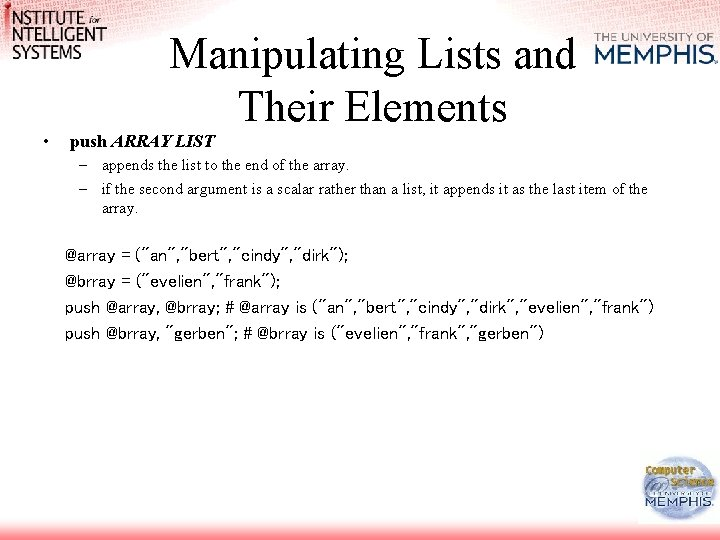Manipulating Lists and Their Elements • push ARRAY LIST – appends the list to the end of the array. – if the second argument is a scalar rather than a list, it appends it as the last item of the array. @array = ("an", "bert", "cindy", "dirk"); @brray = ("evelien", "frank"); push @array, @brray; # @array is ("an", "bert", "cindy", "dirk", "evelien", "frank") push @brray, "gerben"; # @brray is ("evelien", "frank", "gerben")Manipulating Lists and Their Elements pop ARRAY does the opposite of push. it removes the last item of its argument list and returns it. if the list is empty it returns undef. @array = ("an", "bert", "cindy", "dirk"); \$item = pop @array; # \$item is "dirk" and @array is ( "an", "bert", "cindy") shift ARRAY works on the left end of the list, but is otherwise the same as pop. unshift ARRAY LIST puts stuff on the left side of the list, just as push does for the right side.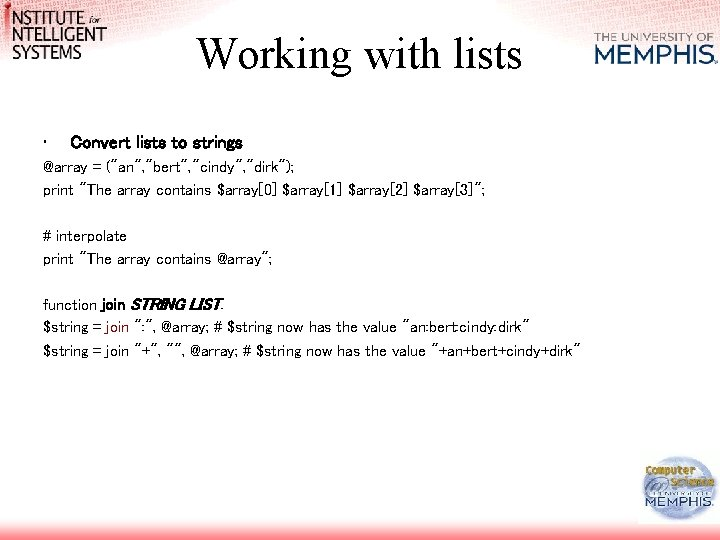Working with lists • Convert lists to strings @array = ("an", "bert", "cindy", "dirk"); print "The array contains \$array \$array \$array \$array"; # interpolate print "The array contains @array"; function join STRING LIST. \$string = join ": ", @array; # \$string now has the value "an: bert: cindy: dirk" \$string = join "+", "", @array; # \$string now has the value "+an+bert+cindy+dirk"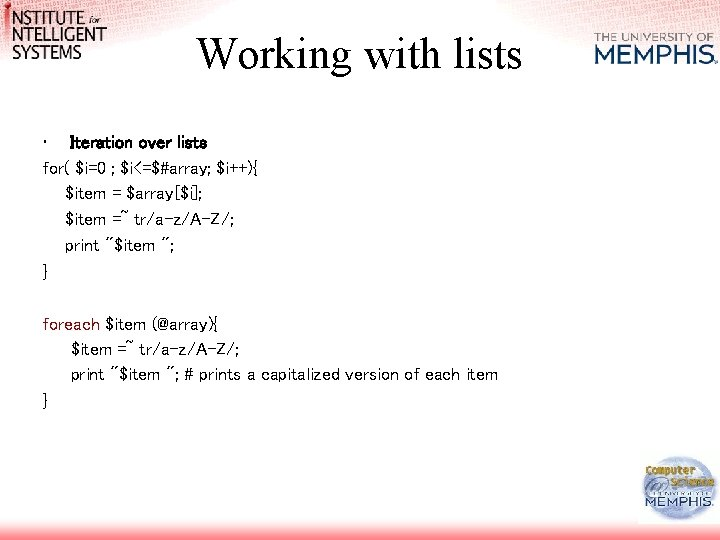Working with lists • Iteration over lists for( \$i=0 ; \$i<=\$#array; \$i++){ \$item = \$array[\$i]; \$item =~ tr/a-z/A-Z/; print "\$item "; } foreach \$item (@array){ \$item =~ tr/a-z/A-Z/; print "\$item "; # prints a capitalized version of each item }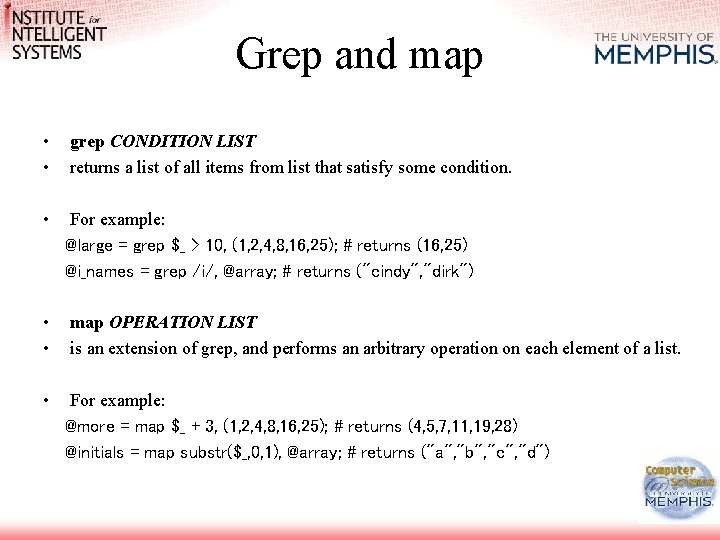Grep and map • • grep CONDITION LIST returns a list of all items from list that satisfy some condition. • For example: @large = grep \$_ > 10, (1, 2, 4, 8, 16, 25); # returns (16, 25) @i_names = grep /i/, @array; # returns ("cindy", "dirk") • • map OPERATION LIST is an extension of grep, and performs an arbitrary operation on each element of a list. • For example: @more = map \$_ + 3, (1, 2, 4, 8, 16, 25); # returns (4, 5, 7, 11, 19, 28) @initials = map substr(\$_, 0, 1), @array; # returns ("a", "b", "c", "d")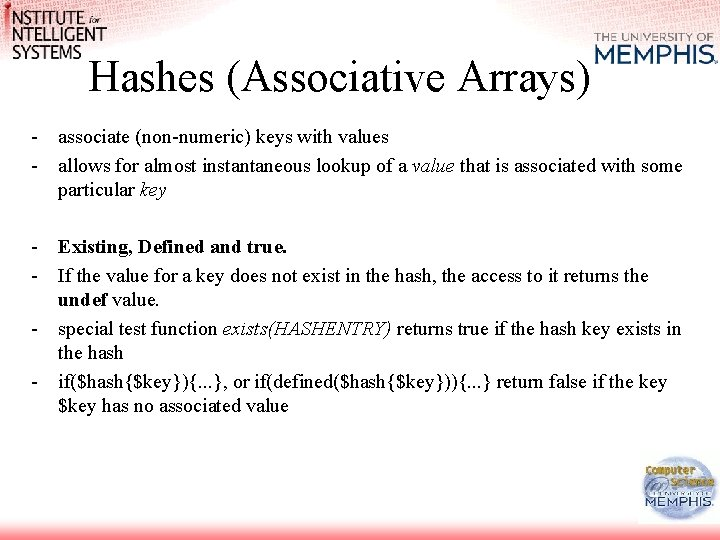Hashes (Associative Arrays) - associate (non-numeric) keys with values - allows for almost instantaneous lookup of a value that is associated with some particular key - Existing, Defined and true. - If the value for a key does not exist in the hash, the access to it returns the undef value. - special test function exists(HASHENTRY) returns true if the hash key exists in the hash - if(\$hash{\$key}){. . . }, or if(defined(\$hash{\$key})){. . . } return false if the key \$key has no associated value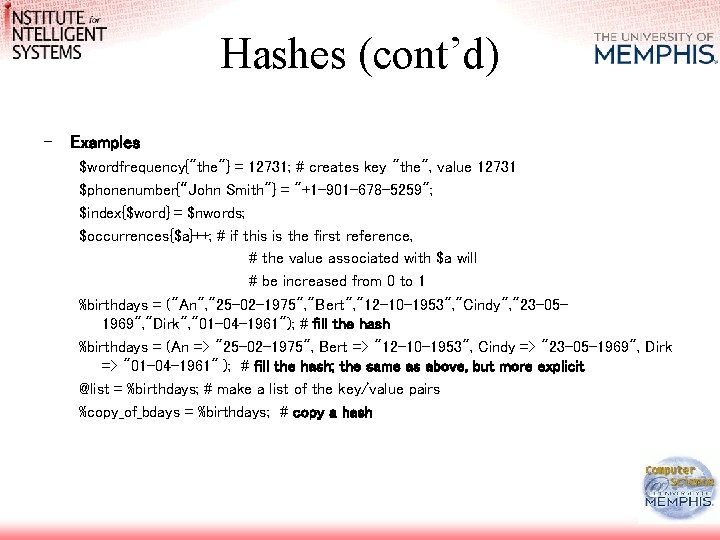Hashes (cont’d) - Examples \$wordfrequency{"the"} = 12731; # creates key "the", value 12731 \$phonenumber{“John Smith"} = "+1 -901 -678 -5259"; \$index{\$word} = \$nwords; \$occurrences{\$a}++; # if this is the first reference, # the value associated with \$a will # be increased from 0 to 1 %birthdays = ("An", "25 -02 -1975", "Bert", "12 -10 -1953", "Cindy", "23 -051969", "Dirk", "01 -04 -1961"); # fill the hash %birthdays = (An => "25 -02 -1975", Bert => "12 -10 -1953", Cindy => "23 -05 -1969", Dirk => "01 -04 -1961" ); # fill the hash; the same as above, but more explicit @list = %birthdays; # make a list of the key/value pairs %copy_of_bdays = %birthdays; # copy a hashOperations on Hashes • keys HASH returns a list with only the keys in the hash. As with any list, using it in a scalar context returns the number of keys in that list. • values HASH returns a list with only the values in the hash, in the same order as the keys returned by keys. foreach \$key (sort keys %hash ){ push @sortedlist, (\$key , \$hash{\$key} ); print "Key \$key has value \$hash{\$key}n"; }Operations on Hashes • reverse the direction of the mapping, i. e. construct a hash with keys and values swapped: %backwards = reverse %forward; • (if %forward has two identical values associated with different keys, those will end up as only a single element in %backwards) - hash slice @birthdays{"An", "Bert", "Cindy", "Dirk"} = ("25 -02 -1975", "12 -10 -1953", "23 -051969", "01 -04 -1961"); each( HASH ) – traverse a hash while ((\$name, \$date) = each(%birthdays)) { print "\$name's birthday is \$daten"; } # alternative: foreach \$key (keys %birthdays)…Multidimensional Data Structures • Perl does not really have multi-dimensional data structures, but a nice way of emulating them, using references \$matrix[\$i][\$j] = \$x; \$lexicon 1{"word"} = \$partofspeech; \$lexicon 2{"word"}{"noun"} = \$frequency; • Array of arrays @matrix = ( # an array of references to anonymous arrays [1, 2, 3], [4, 5, 6], [7, 8, 9] );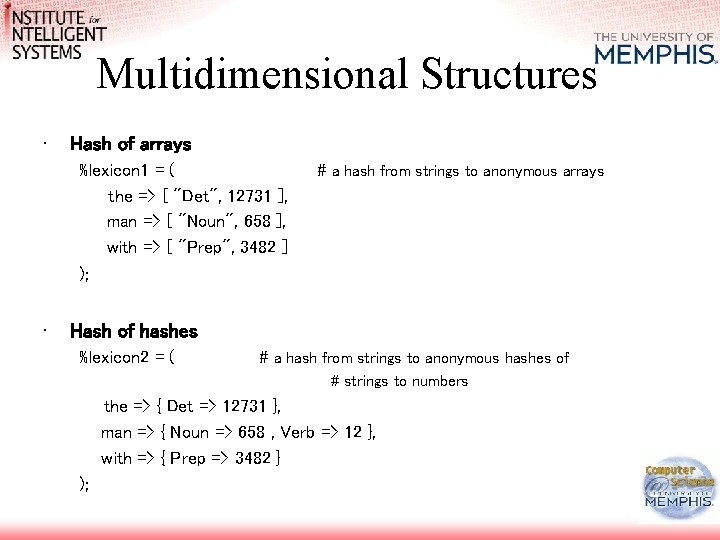Multidimensional Structures • Hash of arrays %lexicon 1 = ( the => [ "Det", 12731 ], man => [ "Noun", 658 ], with => [ "Prep", 3482 ] ); • # a hash from strings to anonymous arrays Hash of hashes %lexicon 2 = ( # a hash from strings to anonymous hashes of # strings to numbers the => { Det => 12731 }, man => { Noun => 658 , Verb => 12 }, with => { Prep => 3482 } );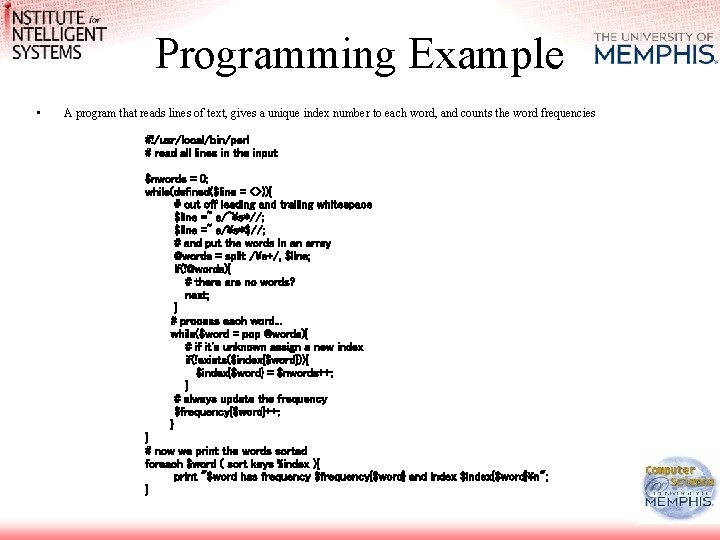Programming Example • A program that reads lines of text, gives a unique index number to each word, and counts the word frequencies #!/usr/local/bin/perl # read all lines in the input \$nwords = 0; while(defined(\$line = <>)){ # cut off leading and trailing whitespace \$line =~ s/^s*//; \$line =~ s/s*\$//; # and put the words in an array @words = split /s+/, \$line; if([email protected]){ # there are no words? next; } # process each word. . . while(\$word = pop @words){ # if it's unknown assign a new index if(!exists(\$index{\$word})){ \$index{\$word} = \$nwords++; } # always update the frequency \$frequency{\$word}++; } } # now we print the words sorted foreach \$word ( sort keys %index ){ print "\$word has frequency \$frequency{\$word} and index \$index{\$word}n"; }A note on sorting • • If we would like to have the words sorted by their frequency instead of by alphabet, we need a construct that imposes a different sort order. sort function can use any sort order that is provided as an expression. the usual alphabetical sort order: sort { \$a cmp \$b } @list; - !! \$a and \$b are placeholders for the two items from the list that are to be compared. Do not attempt to replace them with other variable names. Using \$x and \$y instead will not provide the same effect - a numerical sort order: sort { \$a <=> \$b } @list; - for a reverse sort, change the order of the arguments: sort { \$b <=> \$a } @list; - to sort the keys of a hash by their value instead of by their own identity, substitute the values for the arguments of sort: sort { \$hash{\$b} <=> \$hash{\$a} } ( keys %hash )Basics about Subroutines • Calls to subroutines can be recognized because subroutine names often start with the special character &. sub ask. For. Input { print "Please enter something: "; } # function call &ask. For. Input(); • Tip: put related subroutines in a file (usually with the extention. pm = perl module) and include the file with the command require: # files with subroutines are stored here use lib "C: PERLMYLIBS"; # we will use this file require “ir";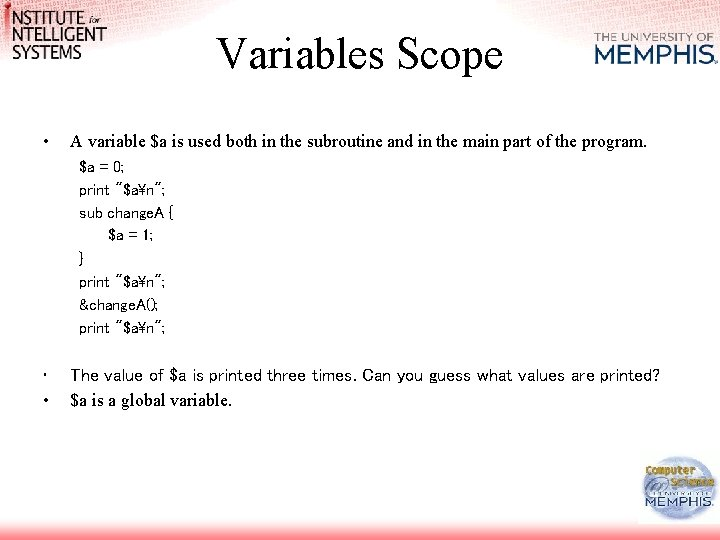Variables Scope • A variable \$a is used both in the subroutine and in the main part of the program. \$a = 0; print "\$an"; sub change. A { \$a = 1; } print "\$an"; &change. A(); print "\$an"; • • The value of \$a is printed three times. Can you guess what values are printed? \$a is a global variable.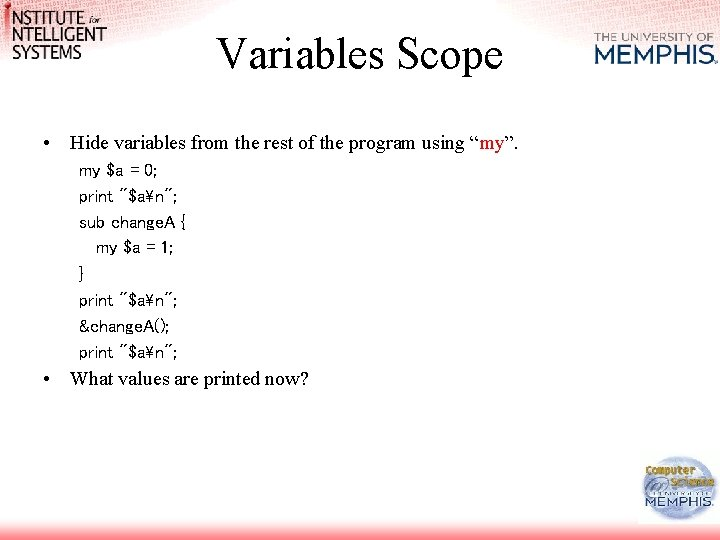Variables Scope • Hide variables from the rest of the program using “my”. my \$a = 0; print "\$an"; sub change. A { my \$a = 1; } print "\$an"; &change. A(); print "\$an"; • What values are printed now?Communication between subroutines and programs • Provide the arguments of the subroutine call: &do. Something(2, "a", \$abc). • Perl converts all arguments to a flat list. This means that &do. Something((2, "a"), \$abc) will result in the same list of arguments as the earlier example. • Access the argument values inside the procedure with the special list @_. – E. g. my(\$number, \$letter, \$string) = @_; # reads the parameters from @_ • A tricky problem is passing two or more lists as arguments of a subroutine. &sub(@a, @b) the subroutine receives the two list as one big list and it will be unable to determine where the first ends and where the second starts. • pass the lists as reference arguments: &sub(@a, @b).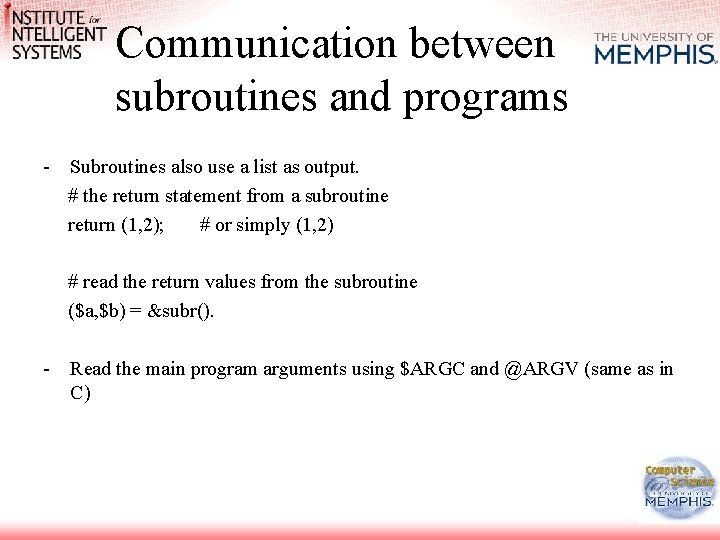Communication between subroutines and programs - Subroutines also use a list as output. # the return statement from a subroutine return (1, 2); # or simply (1, 2) # read the return values from the subroutine (\$a, \$b) = &subr(). - Read the main program arguments using \$ARGC and @ARGV (same as in C)